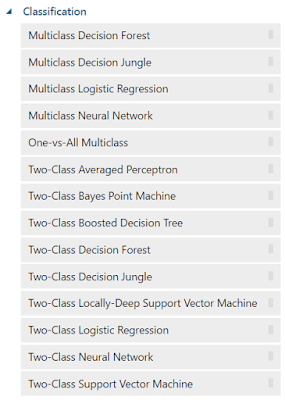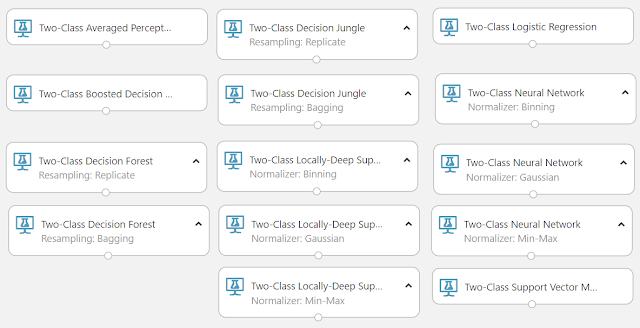## Monday, September 4, 2017

### Azure Machine Learning in Practice: Model Selection

Today, we're going to continue with our Fraud Detection experiment.  If you haven't read our previous posts in this series, it's recommended that you do so.  They cover the Preparation and Data Cleansing phases of the experiment.  In this post, we're going to walk through the model selection process.

In traditional machine learning and data science applications, model selection is a time-consuming process that generally requires a significant amount of statistical background.  Azure Machine Learning completely breaks this paradigm.  As you will see in the next few posts, model selection is Azure Machine Learning requires nothing more than a basic understanding of the problem we are trying to solve and a willingness to let the data pick our model for us.  Let's take a look at our experiment so far.Experiment So Far
We can see that we've already imported our data and decided to use two different imputation methods, MICE and Probabilistic PCA.  Now, we need to select which models we would like to use to solve our problem.  It's important to remember that our goal is predict when a transaction is fraudulent, i.e. has a "Class" value of 1.  Before we do that, we should remember to remove the "Row Number" feature from our dataset, as it has no analytical value.Select Columns in Dataset
Now, let's take a look at our model options.Initialize Model
Using the toolbox on the left side of the Azure Machine Learning Studio, we can work our way down to the "Initialize Model" section.  Here, we have four different types of models, "Anomaly Detection", "Classification", "Clustering" and "Regression".

"Anomaly Detection" is the area of Machine Learning where we try to find things that look "abnormal".  This is an especially difficult task because it requires defining what's "normal".  Fortunately, Azure ML has some great tools that handle the hard work for us.  These types of models are very useful for Fraud Detection in areas like Credit Card and Online Retail transactions, as well Fault Detection in Manufacturing.  However, our training data already has fraudulent transactions labelled.  Therefore, Anomaly Detection may not be what we're looking for.  However, one of the great things about Data Science is that there are no right answers.  Feel free to add some Anomaly Detection algorithms to the mix if you would like.

"Classification" is the area of Machine Learning where we try to determine which class a record belongs to.  For instance, we can look at information about a person and attempt to determine where they are likely to buy a particular product.  This technique requires that we have an initial set of data where already know the classes.  This is the most commonly used type of algorithm and can be found in almost every subject area.  It's not coincidence that our variable of interest in this experiment is called "Class".  Since we already know whether each of these transactions was fraudulent or not, this is a prime candidate for a "Classification" algorithm.

"Clustering" is the area of Machine Learning where we try to group records together to identify which records are "similar".  This is a unique technique belonging to a category of algorithms known as "Unsupervised Learning" techniques.  They are unsupervised in the sense that we are not telling them what to look for.  Instead, we're simply unleashing the algorithm on a data set to see what patterns it can find.  This is extremely useful in Marketing where being able to identify "similar" people is important.  However, it's not very useful for our situation.

"Regression" is the area of Machine Learning where try to predict a numeric value by using other attributes related to it.  For instance, we can use "Regression" techniques to use information about a person to predict their salary.  "Regression" has quite a bit in common with "Classification".  In fact, there are quite a few algorithms that have variants for both "Classification" and "Regression".  However, our experiment only wants to predict a binary (1/0) variable.  Therefore, it would be inappropriate to use a "Regression" algorithm.

Now that we've decided "Classification" is the category we are looking for, let's see what algorithms are underneath it.Classification
For the most part, we can see that there are two types of algorithms, "Two-Class" and "Multiclass".  Since the variable we are trying to predict ("Class") only has two values, we should use the "Two-Class" algorithms.  But which one?  This is the point where Azure Machine Learning really stands out from the pack.  Instead of choosing one, or even a few, algorithms, we can try them all.  In total, there are 9 different "Two-Class Classification" algorithms.  However, in the next post, we'll be looking at the "Tune Model Hyperparameters" module.  Using this module, we'll find out that there are actually 14 distinct algorithms, as some of the algorithms have a few different variations and one of the algorithms doesn't work with "Tune Model Hyperparameters".  Here's the complete view of all the algorithms.Two-Class Classification Algorithms
For those that may have issues seeing the image, here's a list.

Two-Class Averaged Perceptron
Two-Class Boosted Decision Tree
Two-Class Decision Forest - Resampling: Replicate
Two-Class Decision Forest - Resampling: Bagging
Two-Class Decision Jungle - Resampling: Replicate
Two-Class Decision Jungle - Resampling: Bagging
Two-Class Locally-Deep Support Vector Machine - Normalizer: Binning
Two-Class Locally-Deep Support Vector Machine - Normalizer: Gaussian
Two-Class Locally-Deep Support Vector Machine - Normalizer: Min-Max
Two-Class Logistic Regression
Two-Class Neural Network - Normalizer: Binning
Two-Class Neural Network - Normalizer: Gaussian
Two-Class Neural Network - Normalizer: Min-Max
Two-Class Support Vector Machine

A keen observer may notice that the "Two-Class Bayes Point Machine" model was not included in this list.  For some reason, this model cannot used in conjunction with "Tune Model Hyperparameters".  However, we will handle this in a later post.

Hopefully, this post helped shed some light on "WHY" you would choose certain models over others.  We can't stress enough that the path to success is to let the data decide which model is best, not "rules-of-thumb" or theoretical guidelines.  Stay tuned for the next post, where we'll use large-scale model evaluation to pick the best possible model for our problem.  Thanks for reading.  We hope you found this informative.

Brad Llewellyn
Data Scientist
Valorem
@BreakingBI
www.linkedin.com/in/bradllewellyn
llewellyn.wb@gmail.com